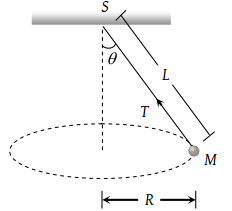In a circus stuntman rides a motorbike in a circular track of radius R in the vertical plane. The minimum speed at highest point of track will be

(1) $\sqrt{2gR}$

(2) 2gR

(3) $\sqrt{3gR}$

(4) $\sqrt{gR}$

Concept Questions :-

Uniform circular motion
High Yielding Test Series + Question Bank - NEET 2020

Difficulty Level:

A block of mass m at the end of a string is whirled round in a vertical circle of radius R. The critical speed of the block at the top of its swing below which the string would slacken before the block reaches the top is

(1) Rg

(2) (Rg)2

(3) R/g

(4) $\sqrt{Rg}$

Concept Questions :-

Uniform circular motion
High Yielding Test Series + Question Bank - NEET 2020

Difficulty Level:

A bucket tied at the end of a 1.6 m long string is whirled in a vertical circle with constant speed. What should be the minimum speed so that the water from the bucket does not spill, when the bucket is at the highest position (Take g = 10 m/s2

(1) 4 m/sec

(2) 6.25 m/sec

(3) 16 m/sec

(4) None of the above

Concept Questions :-

Uniform circular motion
High Yielding Test Series + Question Bank - NEET 2020

Difficulty Level:

A 1 kg stone at the end of 1 m long string is whirled in a vertical circle at constant speed of 4 m/sec. The tension in the string is 6 N, when the stone is at (g = 10 m/sec2

(1) Top of the circle

(2) Bottom of the circle

(3) Half way down

(4) None of the above

Concept Questions :-

Uniform circular motion
High Yielding Test Series + Question Bank - NEET 2020

Difficulty Level:

The tension in the string revolving in a vertical circle with a mass m at the end which is at the lowest position

(1) $\frac{m{v}^{2}}{r}$

(2) $\frac{m{v}^{2}}{r}-mg$

(3) $\frac{m{v}^{2}}{r}+mg$

(4) mg

Concept Questions :-

Uniform circular motion
High Yielding Test Series + Question Bank - NEET 2020

Difficulty Level:

A coin, placed on a rotating turn-table slips, when it is placed at a distance of 9 cm from the centre. If the angular velocity of the turn-table is trippled, it will just slip, if its distance from the centre is

(1) 27 cm

(2) 9 cm

(3) 3 cm

(4) 1 cm

Concept Questions :-

Uniform circular motion
High Yielding Test Series + Question Bank - NEET 2020

Difficulty Level:

A body of mass 0.4 kg is whirled in a vertical circle making 2 rev/sec. If the radius of the circle is 2 m, then tension in the string when the body is at the top of the circle, is

(1) 41.56 N

(2) 89.86 N

(3) 109.86 N

(4) 122.4 N

Concept Questions :-

Uniform circular motion
High Yielding Test Series + Question Bank - NEET 2020

Difficulty Level:

A bucket full of water is revolved in vertical circle of radius 2m. What should be the maximum time-period of revolution so that the water doesn't fall off the bucket

(1) 1 sec

(2) 2 sec

(3) 3 sec

(4) 4 sec

Concept Questions :-

Uniform circular motion
High Yielding Test Series + Question Bank - NEET 2020

Difficulty Level:

A tube of length L is filled completely with an incompressible liquid of mass M and closed at both the ends. The tube is then rotated in a horizontal plane about one of its ends with a uniform angular velocity ω. The force exerted by the liquid at the other end is

(1) $\frac{ML{\omega }^{2}}{2}$

(2) $ML{\omega }^{2}$

(3) $\frac{ML{\omega }^{2}}{4}$

(4) $\frac{M{L}^{2}{\omega }^{2}}{2}$

High Yielding Test Series + Question Bank - NEET 2020

Difficulty Level:

A string of length L is fixed at one end and carries a mass M at the other end. The string makes 2/π revolutions per second around the vertical axis through the fixed end as shown in the figure, then tension in the string is(1) ML

(2) 2 ML

(3) 4 ML

(4) 16 ML